# Flight Price prediction

This Article is generally on ‘Prediction on flight price’ a hackathon hosted on machinehack.com takes you through each and every step in detail and helps you understand the whole ML model building process . So, let’s get started.

#### Problem Statement

Flight ticket prices can be something hard to guess, today we might see a price, check out the price of the same flight tomorrow, it will be a different story. We might have often heard travelers saying that flight ticket prices are so unpredictable. As data scientists, we are gonna prove that given the right data anything can be predicted. Here you will be provided with prices of flight tickets for various airlines between the months of March and June of 2019 and between various cities.

#### Datasets

We will be using two datasets — Train data and Test data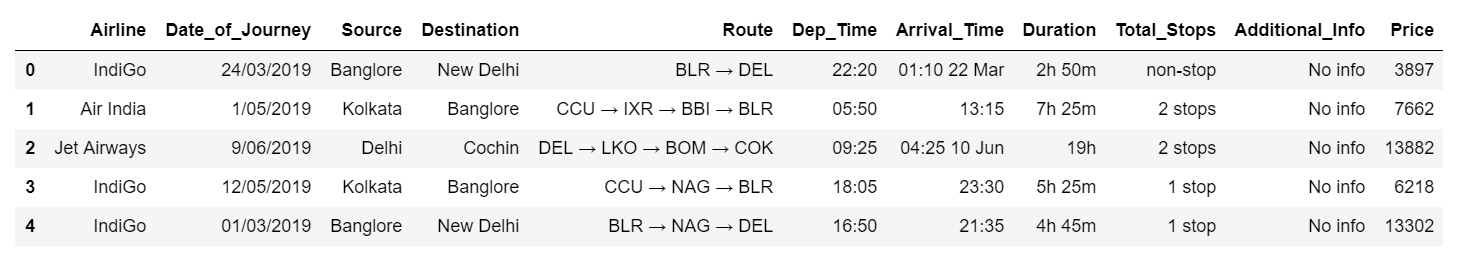Screenshot of the Training data (10683 rows): Training data refers to that portion of data used to fit a model.

Training data is combination of both categorical and numerical also we can see some special character also being used because of which we have to do data Transformation on it before applying it to our model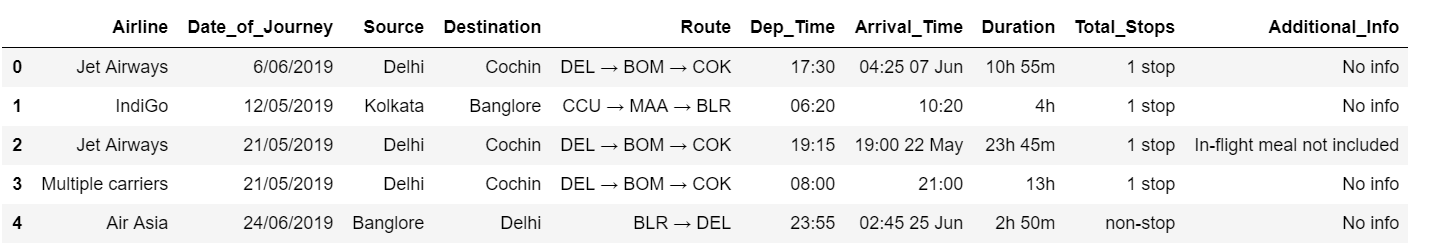Screenshot of the Test data ( 2671 rows)

The test data is similar to the training data set, minus the ‘Price’ column (To be predicted using the model).

#### Python Coding

Step 1: Import the relevant libraries in Python.

Step 2: Import Train and Test data sets and append them

Appending of the data set is done to work together with both train and test at a same time and don,t have to make changes separately.After we apply the transformation then we can separate them again into test and train

Step 3: Feature Generation

In this step we mainly work on the data set and do some transformation like creating different bins of particular columns ,clean the messy data so that it can be used in our ML model . This step is very important because for a high prediction score you need to continuously make changes in it

Date_of_Journey:

In the column ‘Date_of_Journey’, we can see the date format is given as dd/mm/yyyy and as you can see the datatype is given as object So there is two ways to tackle this column, either convert the column into Timestamp or divide the column into date,Month ,Year. Here , i am splitting the columns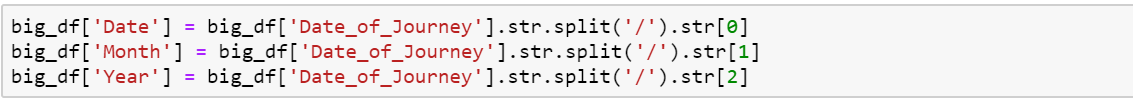Date_of_Journey split into 3 variables (Date, Month, Year )

Arrival_Time:

In the column ‘Arrival_Time’,if we see we have combination of both time and month but we need only the time details out of it so we split the time into ‘Hours’ and ‘Minute’.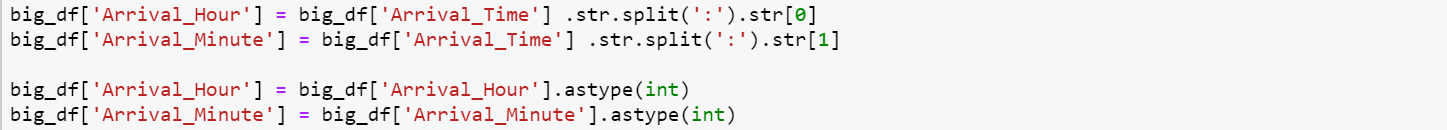Arrival_Time split into 2 variables (Hour, Minute)

Total_Stops:

This column is combination of number and a categorical variable like ‘1 stop’ . So we need only the number details from this column so we split that and take the number details only also we change the ‘non stop’ into ‘0 stop’ and convert the column into integer type

Dep_Time:

As same as ‘Arrival_time’ .we split this column also in hour and minute and convert it into integer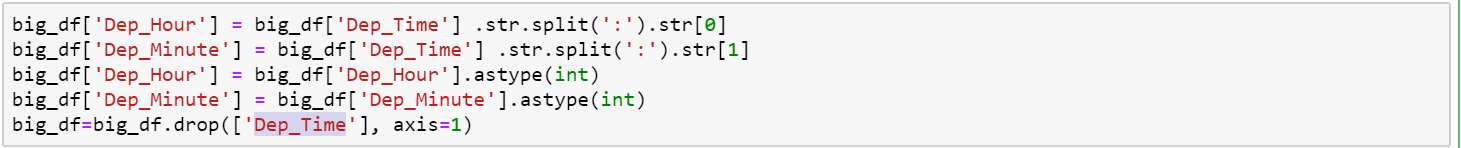Dep_Time split into 2 variables (Hour, Minute)

Route:

The ‘Route’ columns mainly tell us that how many cities they have taken to reach from source to destination .This column is very important because based on the route they took will directly effect the price of the flight So We split the Route column to extract the information .Regarding the ‘Nan’ values we replace those ‘Nan’ values with ‘None’ .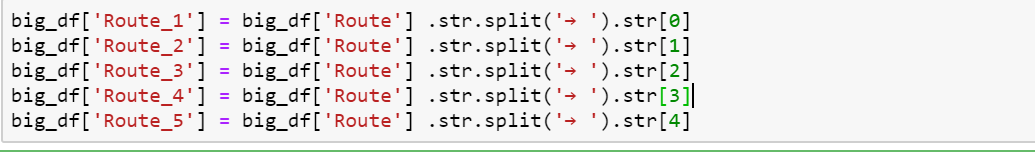Route split into 5 variables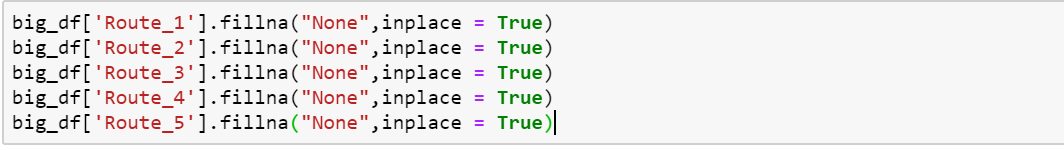Replacing the Nan values with ‘None’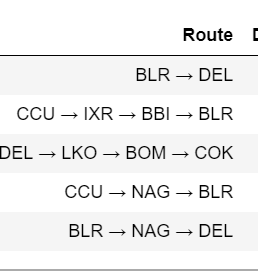Before splitting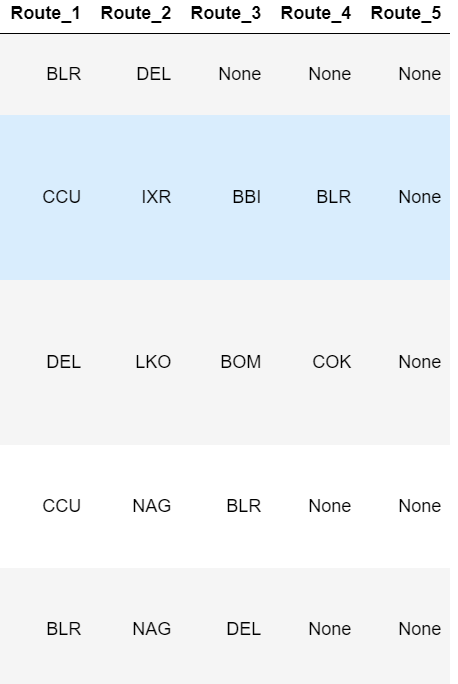After splitting

Step 4: Prepare categorical variables for model using label encoder

To convert categorical text data into model-understandable numerical data, we use the Label Encoder class. So all we have to do, to label encode a column is import the LabelEncoder class from the sklearn library, fit and transform the column of the data, and then replace the existing text data with the new encoded data.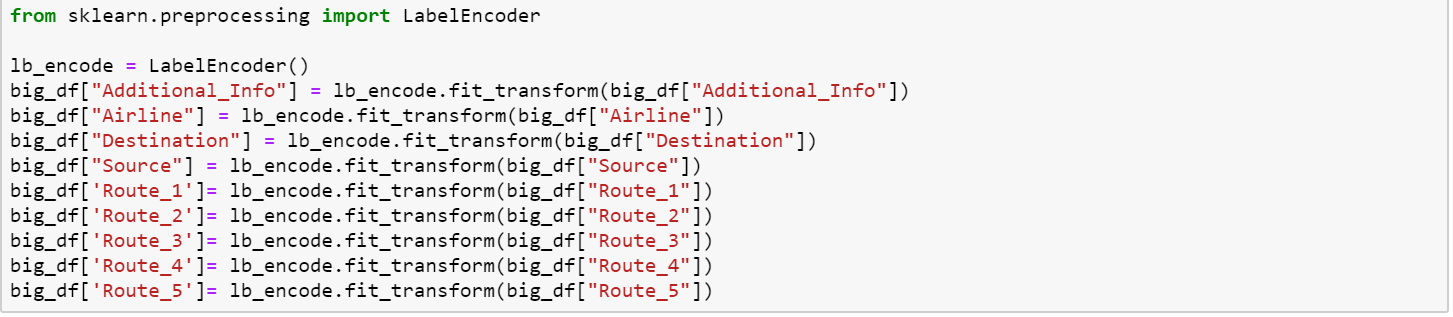Label encoding of Categorical variables

Step 5 : Divide the data set into test and train

Now that all our data is numerical after label encoding so we split the data into test and train and drop the price column from the test set because we have to predict the price with our test data set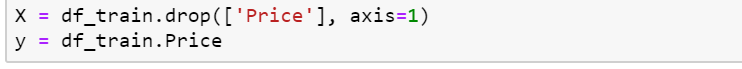X — independent variables; y — dependent variable

Step 6: Build Model

The goal in this step is to develop a benchmark model that serves us as a baseline, upon which we will measure the performance of a better and more tuned algorithm. We are using different Regression Technique and comparing them to see which algorithm is giving better performance then other and At the end we will combine all of them using Stacking and see how our model is predicting

1. Linear Regression :You can check the below link for more details on the Regression Technique that we are using

RMSE( Root Mean Square Error):3238.316987636252

2. Ridge Regression:You can check the below link for more details on the Regression Technique that we are using

RMSE( Root Mean Square Error):3238.153926834792

3. Lasso Regression:You can check the below link for more details on the Regression Technique that we are using

RMSE( Root Mean Square Error):3273.005929514414

4. Elastic Net Regularization:You can check the below link for more details on the Regression Technique that we are using

RMSE( Root Mean Square Error):3238.296057360342

5.Extreme Gradient Boosting (XGBoost):You can check the below link for more details on the Regression Technique that we are using

RMSE( Root Mean Square Error):1281.0225332975244

6.Light GBM:You can check the below link for more details on the Regression Technique that we are using

RMSE( Root Mean Square Error):1747.2331238078746

7. STACKING:

Stacking is an ensemble learning technique that combines multiple classification or regression models via a meta-classifier or a meta-regressor. The base level models are trained based on a complete training set, then the meta-model is trained on the outputs of the base level model as features.

RMSE( Root Mean Square Error):1372.627469

From the above different Regression Technique we can see XGboost is performing really good in regards to all .Finally we will use this to predict our test data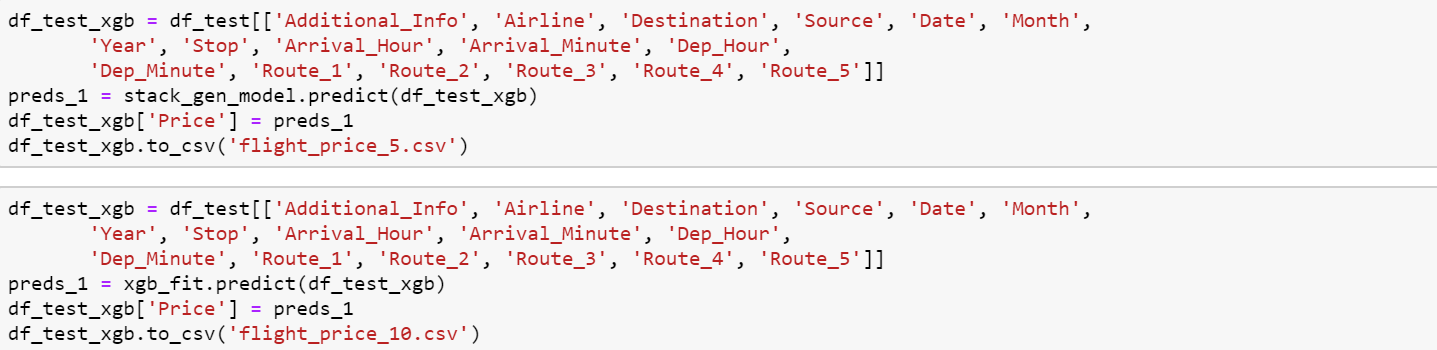Export it into a csv file and submit it

Final Word

In this type of problem Feature Engineering is the most crucial think . You can see how we have handled the categorical and numerical data and also how we build different ML model on the same dataset . We also check the RMSE score of each model so that we can understand how it should perform in our test dataset . At last You can also further improve the Model by Tunning different parameters which are being used in the model . Please let me know your thoughts about this article and do comment if you face any issues.

As always, I welcome feedback and constructive criticism. I can be reached on snehanshu.sengupta1991@gmail.com

Sources :

### Get your own blog published on C2E Blog

Do you want to write for CodeToExpress? We would love to have you as a technical writer. Send us an email with a link to your draft at codetoexpress@gmail.com